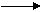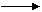Chemistry Paper 3,May/June 2014
 Questions: 1 2 3 Main
Weakness/Remedies
Strength

Question 1

1.         A is a solution of hydrochloric acid.
B is a solution containing 2.45 g of anhydrous sodium trioxocarbonate (IV) in
250 cm3 of solution.

(a)        Put A into the burette and titrate it against 20.0 cm3 or 25.0cm3 portion
of B using methyl orange as indicator.  Repeat the exercise to obtain                                               consistent titres.  Tabulate your burette readings and calculate the
average volume of A used.
The equation for the reaction involved in the titration is:
Na2CO3(aq) + 2HC1(aq)2NaC1(aq) + H2O(1) + CO2(g)
[10 marks]

(b)        From your results and the information provided, calculate the:

(i)         concentration of B in moldm-3;
(ii)        concentration of A in moldm-3;
(iii)       concentration of A in moldm-3;
(iv)       volume of the gas evolved in the reaction at s.t.p.
[H = 1.00; C = 12.0; O = 16.0; Na = 23.0; C1= 35.6;
Molar Volume = 22.4 dm3 mol-1]
[11 marks]

_____________________________________________________________________________________________________
OBSERVATION

This question was based on acid-base titration.  It was attempted by majority of the           candidates and their performance was good.

In part (a), candidates correctly tabulated their burette readings and calculated the             average volume of acid used using concordant tire values.  However, some candidates
lost marks due to alteration of their titre values and wrong averaging of titres.

In the part (b), majority of the candidates correctly calculated the concentration of
A and B in moldm-3.  However, some candidates lost marks due to wrong substitution      and units.   In part (b) (iv) only a few candidates correctly calculated the volume of CO2
evolved in the reaction at s.t.p.

Question 1

(a)        (i)         Titrations:  4 x 2 sets
Averaging(Vcm3)

Na2CO3(aq) + 2HC1(aq)2NaC1(aq) + H2O + CO2(g)

(b)        (i)         Conc. of B in moldm-3
Molar mass (Na2CO3) = (2 x 23) + 12 + (3 x 16) + 106 gmol-1
Conc. of B (Na2CO3)  =         2.45           =   0.0925 mol dm-3
106 x 0.25
Alt. Method
250 cm 3 of solution contained 2.45g of Na2CO3
1000 cm 3 of solution contained  2.45 x 1000
250

=              2.45x4
=          9.80g
M(Na2CO3)  =  106gmol-1
Conc. B(Na2 CO3)   =   9.80      =0.0925 moldm-3
106
Conc. of A in moldm-3
From reaction equation
CA VA          =     2                                               CA =   2 x CB x VB
CBVB                   1                                                       VB

CA =   2 x 0.0925 x 20/25    =  a moldm-3 (correct evaluation to 3 sig. figs)
VA

Conc. of A in gdm-3
M(HC) = 1 + 35.5      =  36.5gmol-1

Conc. of A in (gdm-3)  = a x molar mass (HC1)
= a x 36.5
= b gdm-3

(iv)       Volume of the gas evolved
From reaction equation
1 mole of Na2CO3  =  1 mole of CO2 at stp
n(CO2)  =   n (Na2CO3)  =  0.0925 mole
… Vco2    =    n x Vm
=  0.0925 x 22.4dm3
=  2.07dm3 at stp
… Vco2    liberated in 25 cm3 of Na2CO3 will be 2,07 x 25 x   25
1000
= 0.0518 dm3/51.80 cm3 at stp